# The Definitive Guide to Using Dates and Times in Excel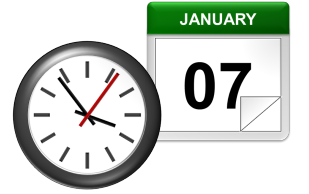Dates and times are two of the most common data types people work with in Excel, but they are also possibly the most frustrating to work with, especially if you are new to Excel and still learning. This is because Excel uses a serial number to represent the date instead of a proper month, day, or year, nevermind hours, minutes, or seconds. It’s made more complicated by the fact that dates are also days of the week, like Monday or Friday, even though Excel doesn’t explicitly store that information in the cells. Here is the definitive guide to working with dates and times in Excel…

## How Excel Stores Dates

The source of most of the confusion around dates and times in Excel comes from the way that the program stores the information. You’d expect it to remember the month, the day, and the year for dates, but that’s not how it works…

Excel stores dates as a serial number that represents the number of days that have taken place since the beginning of the year 1900. This means that January 1, 1900 is really just a 1. January 2, 1900 is 2. By the time we get all the way to the present decade, the numbers have gotten pretty big… September 10, 2013 is stored as 41527.

Importantly, any date before January 1, 1900 is not recognized as a date in Excel. There are no “negative” date serial numbers on the number line.

It seems confusing, but it makes it a lot easier to add, subtract, and count days. A week from September 10, 2013 (September 17, 2013), is just 41527 + 7 days, or 41534.

## How Excel Stores Times

Excel stores times using the exact same serial numbering format as with dates. Days start at midnight (12:00am or 0:00 hours). Since each hour is 1/24 of a day, it is represented as that decimal value: 0.041666…

That means that 9:00am (09:00 hours) on September 10, 2013 will be stored as 41527.375.

When a time is specified without a date, Excel stores it as if it occurred on January 0, 1900. In other words, 3:00pm (15:00 hours) is stored as 0.625. This can make doing math for time-only values (that have no date) challenging, since subtracting 6 hours (6:00)  from 3:00am (03:00 hours) will become negative and count as an error: 0.125 – 0.25 = -0.125, which is displayed as #########.

Minutes and seconds in Excel work the same way as hours…

A minute is 1/60 of an hour, which is 1/24 of a day, or 1/1440 of a day in total, which calculates to 0.00069444…

A second is 1/60 of an minute, which is 1/60 of an hour, which is 1/24 of a day, or 1/86400 of a day in total, which calculates to 0.00001157407…

## Working with Dates and Times

### DATE() and TIME()

Serial numbers aren’t all that intuitive to use. Fortunately, Excel has a set of functions to make it easier to find and use dates and times, starting with DATE and TIME. The syntax is as follows:

`=DATE(year, month, day)`
`=TIME(hours, minutes, seconds)`

For both functions, specify the year, month, and day, or hours, minutes, and seconds as numbers. For example, September 10, 2013 can be entered as:

`=DATE(2013,9,10)`

It will be stored as 41527, which means that it is technically storing 12:00am on September 10, 2013.

For times, 6:00pm (18:00 hours) can be entered as:

`=TIME(18,0,0)`

It will be stored as 0.75, which means that it is technically storing 6:00pm on January 0, 1900.

If we want to represent a specific time and date, we can add the two functions together. For example, 6:00pm (18:00 hours) on September 10, 2013 can be entered as:

`=DATE(2013,9,10)+TIME(18,0,0)`

It will be calculate as 41527.75, which means Excel is storing exactly the date we want…

## Additional Date and Time Setting Functions

Excel has a few additional functions to make declaring dates easier.

### TODAY()

The TODAY function always returns the current date’s serial number. The TODAY function is just entered as:

`=TODAY()`

This article was written at 6:30pm (18:30 hours) on September 24, 2013, and the TODAY function calculated to 41541.  That means that it is technically storing 12:00am on September 24, 2013.

### NOW()

A similar function called NOW always returns the current date and time’s serial number. The NOW function is just entered as:

`=NOW()`

Again,  at 6:30pm (18:30 hours) on September 24, 2013, the function calculated to 41541.77081333… NOW stores the exact time and date, down to the second.

### EDATE() and EOMONTH()

The EDATE function gives the date the specified number of months away from the input date. The EOMONTH function gives the date of the last day of the month. It can do so for the current month or a number of months in the future or the past. The syntax for each is as follows:

`=EDATE(start_date, months)`
`=EOMONTH(start_date, months)`

The start_date can be any date-formatted cell reference or date serial number.

The months field can be any number, though only the integer value will be used (e.g. it treats 2.8 as 2).

The EDATE and EOMONTH functions strip the time value from the date. For example, For example, if cell A1 stores September 10, 2013, we can get the value 2 months ahead as follows:

`=EDATE(A1,2)`

Returns 12:00am (0:00 hours) on November 10, 2013, or 41588. This function works even though the months have different numbers of days (September and November have 30, October has 31).

`=EOMONTH(A1,2)`

Returns 12:00am (0:00 hours) on November 30, 2013, or 41588. Again, this function works even though the months have different numbers of days.

### WORKDAY()

Occasionally, it may be useful to count ahead based on work-days (Monday-Friday) instead of all 7 days of the week… For that, Excel has provided WORKDAY. The syntax for WORKDAY is as follows:

`=WORKDAY(start_date, days, [holidays])`

The start_date is as above.

The days input is the number of workdays ahead (or behind) of the present day you would like to move.

The [holidays] input is optional, but lets you disqualify specific days (like Thanksgiving or Christmas, for example), which might otherwise fall during the work week. These are date serial numbers provided in an array bounded by brackets: { }. To specify multiple holidays, the dates must be held in cells – it is not possible to put multiple DATE functions in an array.

For example, let’s find the date 6 work days before 6:00pm (18:00 hours) on September 10, 2013 (stored in cell A1). Monday, September 2nd is Labor Day, so let’s include that as a holiday:

`=WORKDAY(A1,-6,DATE(2013,9,2))`

Returns 12:00am (0:00 hours) on August 30, 2013, or 41516. (Note that the function strips the time portion of the date.)

### WORKDAY.INTL() (Excel 2010 and newer)

For newer versions of Excel (2010 and later), there is another version of WORKDAY called WORKDAY.INTL. WORKDAY.INTL works just like WORKDAY, but it adds the ability to customize the definition of the “weekend”. The syntax for WORKDAY.INTL is as follows:

`=WORKDAY.INTL(start_date, days, [weekend], [holidays])`

The start_date, days, and [holidays] inputs work just like the normal WORKDAY function.

The [weekend] input has the following options:## Retrieving Dates in Excel

### DAY(), MONTH(), and YEAR()

Now we know how define dates, but we still need to be able to work with them. Serial numbers don’t make it easy to extract months, years, and days, nevermind hours, minutes, and seconds. That’s why Excel has specific functions for pulling out each of these values. For working with the calendar, there is DAY, MONTH, and YEAR. The syntax is simple:

`=DAY(serial_number)`
`=MONTH(serial_number)`
`=YEAR(serial_number)`

The serial_number in each can be any date-formatted cell reference. For example, if cell A1 stores September 10, 2013, we can use each of the formulas in turn:

`=DAY(A1)`

Returns 10 as a numeric value.

`=MONTH(A1)`

Returns as a numeric value.

`=YEAR(A1)`

Returns 2013 as a numeric value.

We could have also given the direct serial number for September 10, 2013:

`=DAY(41527)`

Returns 10 as a numeric value.

## Retrieving Times in Excel

### HOUR(), MINUTE(), and SECOND()

For times, the process is very similar. Excel has function to retrieve the hours, minutes, and seconds from a time stamp, conveniently named HOUR, MINUTE, and SECOND. The syntax is identical:

`=HOUR(serial_number)`
`=MINUTE(serial_number)`
`=SECOND(serial_number)`

The serial_number in each can be any time/date-formatted cell reference. For example, if A1 stores 6:15:30pm (18:15 hours, 30 seconds) on September 10, 2013, we can use each of the formulas in turn:

`=HOUR(A1)`

Returns 18 as a numeric value.

`=MINUTE(A1)`

Returns 15 as a numeric value.

`=SECOND(A1)`

Returns 30 as a numeric value.

We could have also given the direct serial number for  6:15:30pm (18:15 hours, 30 seconds) on September 10, 2013:

`=SECOND(41527.7607638889)`

Returns 30 as a numeric value.

### WEEKDAY() and WEEKNUM()

Dates don’t just have month and year information. They also encode indirect information… September 10, 2013 happens to also be a Tuesday. Excel has a few of functions to work with the week aspect of dates: WEEKDAY and WEEKNUM. The syntax is as follows:

`=WEEKDAY(serial_number, [return_type])`
`=WEEKNUM(serial_number, [return_type])`

The serial_number in each can be any date-formatted cell reference. Since [return_type] is optional, each function assumes that each week starts on Sunday. If cell A1 stores September 10, 2013 (a Tuesday), we can use each of the formulas in turn:

`=WEEKDAY(A1)`

Returns 3, since Tuesday is the 3rd day of a week that starts on Sunday.

`=WEEKNUM(A1)`

Returns 37, since September 10, 2013 is in the 37th week of 2013, when you start counting weeks from Sunday.

The [return_type] allows you to specify a different default week arrangement. You could let the week start on Monday and run until Sunday, or Saturday until Friday, for example. Excel is annoying, however, and makes the entry different for WEEKDAY and WEEKNUM. The full list of options for WEEKDAY is as follows:Options 2 and 11 are functionally the same – the first is just there for backwards compatibility with earlier versions of Excel.

The full list of options for WEEKNUM is as follows:## Counting and Tracking Dates

Dates can be added and subtracted like normal numbers because they’re stored as serial numbers. That lets you count the days between two different dates. Sometimes, though, you need to count by a different metric.

### NETWORKDAYS()

Above, we learned about WORKDAY, which lets you move back and forth a set number of workdays, ignoring weekends and holidays. But what if you need to measure the number of workdays between two dates? For that, Excel provides NETWORKDAYS. The formula syntax is as follows:

`=NETWORKDAYS(start_date, end_date, [holidays])`

The start_date and end_date can be any date-formatted cell reference.

The [holidays] input is optional, but lets you disqualify specific days (like Thanksgiving or Christmas, for example), which might otherwise fall during the work week. These are date serial numbers provided in an array bounded by brackets: { }. To specify multiple holidays, the dates must be held in cells – it is not possible to put multiple DATE functions in an array.

For example, if A1 contains 6:00pm (18:00 hours) on September 10, 2013 and B1 contains 9:00am (9:00 hours) on December 2, 2013, we can use NETWORKDAYS to find the number of workdays between the two dates.

Let’s exclude Columbus Day (October 14, 2013), Veterans Day (November 11, 2013), and Thanksgiving Day (November 28, 2013) as holidays. To do so, we have to store those dates in other cells. Let’s put them in C1, C2, and C3:

`=DATE(2013,10,14)`
`=DATE(2013,11,11)`
`=DATE(2013,11,28)`

Now, we can combine them in the function:

`=NETWORKDAYS(A1,B1,C1:C3)`

The function returns 57 as a numeric value.

### NETWORKDAYS.INTL() (Excel 2010 and newer)

For newer versions of Excel (2010 and later), there is another version of NETWORKDAYS called NETWORKDAYS.INTLNETWORKDAYS.INTL works just like NETWORKDAYS, but it adds the ability to customize the definition of the “weekend”. The syntax for NETWORKDAYS.INTL is as follows:

`=NETWORKDAYS.INTL(start_date, end_date, [weekend], [holidays])`

The start_dateend_date, and [holidays] inputs work just like the normal WORKDAY function.

The [weekend] input has the following options:### YEARFRAC()

Sometimes it’s useful to measure how much time has passed in years, but subtracting the YEAR function will only round down to the nearest full year. YEARFRAC takes two dates and provides the portion of the year between them. The syntax is as follows:

`=YEARFRAC(start_date, end_date, [basis])`

The start_date and end_date can be any date-formatted cell reference.

The [basis] input is optional, but lets you specify the “rules” for measuring the difference. Most of the time, you’ll want to use option 1, but here is the full list:For example, if A1 contains September 10, 2013 and B1 contains December 2, 2013, we can use YEARFRAC to find the decimal portion of a year between the two dates:

`=YEARFRAC(A1,B1,1)`

Returns 0.227397260273973.

### DATEDIF() (Undocumented Function)

The YEARFRAC function gives you the difference between dates as a fraction of a year, but sometimes you need more control. There is a powerful hidden function in Excel called DATEDIF that can do much more. It can tell you the number of years, months, or days between two dates. It can also track based on only partial inputs, ignoring years or months when calculating days. The syntax for DATEDIF is as follows:

`=DATEDIF(start_date, end_date, unit)`

The start_date and end_date can be any date-formatted cell reference.

The unit input asks you to specify a string that represents the type of output you want. This is slightly cumbersome, since you must wrap the input in quotes (” “).

For example, if A1 contains September 10, 2012 and B1 contains December 2, 2013, we can use DATEDIF to find the number of full years between the two dates:

`=DATEDIF(A1,B1,"Y")`

Returns 1 as a numeric value.

Using the same start_date and end_date inputs, here are the output possibilities for DATEDIF using different unit parameters: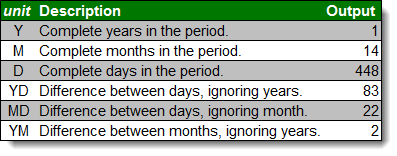Every time, the unit must be put in quotes (e.g. “Y” or “MD”).

## Converting Dates and Times from Text

### DATEVALUE() and TIMEVALUE()

All of the above functions work perfectly with date-formatted serial numbers in Excel. Unfortunately, dates and times are often imported into worksheets as text. Most of the assorted functions like MONTH and HOUR are reasonably intelligent about converting on the fly. Occasionally it’s useful to build a date value through concatenation. The two functions Excel provides for this purpose are DATEVALUE and TIMEVALUE. The syntax for each is as follows:

`=DATEVALUE(date_text)`
`=TIMEVALUE(time_text)`

The date_text and time_text accept any text string that looks like a date or time.

This is how DATEVALUE responds to various date_text inputs:This is how TIMEVALUE responds to various time_text inputs:## Converting Dates and Times to Text

### TEXT()

Getting data converted to dates and times is great, but you may also need to get it back out. Sometimes, you need a special format. Other times, you need to look for a date in a text string, and have to match using string tools like FIND and SEARCH. There is one master function for converting dates and times to text strings in Excel, called TEXT. The syntax for TEXT is as follows:

`=TEXT(value, format_text)`

The value can be any date or time-formatted cell reference.

The format_text input has a large number of options, summarized here: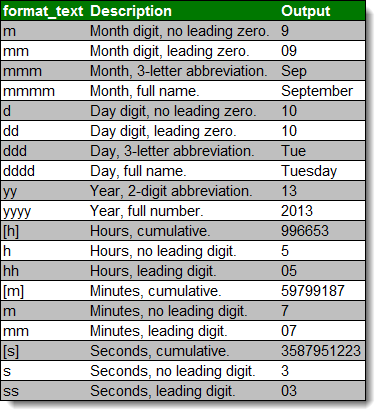The outputs can be combined with simple formatting characters to produce standard date formats. Using the date 5:07:03am (05:07 hours, 3 seconds) on September 10, 2013, here are examples of possible outputs: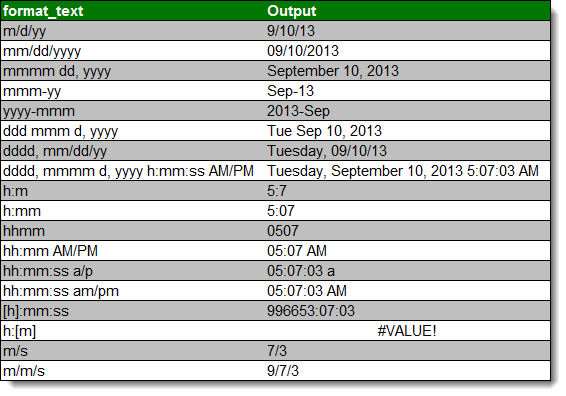## A Common Problem

One issue people frequently run into is that Excel occasionally misinterprets text fields as dates. An example is here: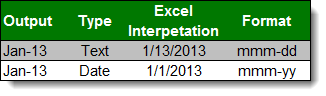Be careful when entering dates, especially if you are importing from other data sources, to make sure that your “Jan-13” is being stored as January 1, 2013 and not January 13, 2013!